Study P3 Mathematics Angles - Geniebook# Angles

1. Understanding angles
2. Identifying angles

## 1. What is an Angle?

An angle is formed when two lines meet at a point.The angle is usually measured in degrees and is represented by the symbol “$$\angle$$”.

Question 1:

Look at the figure below.How many angles are there inside the figure?

1. 5
2. 6
3. 7
4. 4

Solution:There are 6 angles in this figure.

General Rule: Number of corners $$=$$ Number of angles

(2) 6

Question 2:

Look at the figure below.How many angles are there inside the figure?

1. 6
2. 8
3. 10
4. 4

Solution:(2) 8

## 2. Identifying Angles

There are three main types of angles:

1. Acute Angle: angle that is less than $$90^\circ$$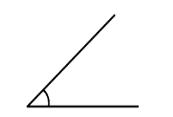1. Right Angle: angle that is exactly $$90^\circ$$1. Obtuse Angle: angle that is greater than $$90^\circ$$ but smaller than $$180^\circ$$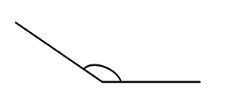What do you notice about the angles on a straight line?It is $$180^\circ!$$

There are 2 right angles on a straight line.

A right angle is $$90^\circ$$

$$90^\circ + 90^\circ = 180^\circ$$

Hence, the angles on a straight line add up to $$180^\circ$$.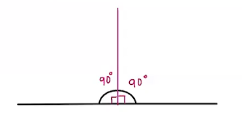Question 1:

Look at the figure below: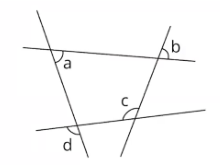__________ and __________ are acute angles.

Solution:

To identify if an angle is acute, we place a ruler on one of the lines of an angle.

If the second line of the angle lies within the ruler, it is an acute angle.

If the second line of the angle does not lie within the ruler, it is an obtuse angle.Since $$\angle a$$ and $$\angle b$$ lies within the ruler, they are less than $$90^\circ$$. Hence, they are acute angles.Since $$\angle c$$  does not lie within  the ruler,  it is more than $$90^\circ$$. Hence, it is an obtuse angle.

$$\angle a$$ and $$\angle b$$

Question 2:

Look at the figure below: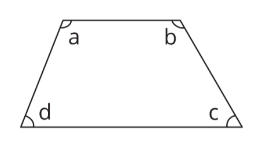Which of the following angles are smaller than a right angle?

1. $$\angle a$$ and $$\angle b$$
2. $$\angle b$$ and $$\angle c$$
3. $$\angle c$$ and $$\angle d$$
4. $$\angle a$$ and $$\angle d$$

Solution:

After placing the ruler on one line of $$\angle a$$, we see that the second line forming $$\angle a$$ does not lie within the ruler. Hence, $$\angle a$$ is an obtuse angle. It is the same for $$\angle b$$After placing the ruler on one line of $$\angle c$$, we see that the second line forming $$\angle c$$ lies within the ruler. Hence, $$\angle c$$ is an acute angle. It is the same for $$\angle d$$.Acute angles are angles that are smaller than a right angle.

(3) $$\angle c$$ and $$\angle d$$

Question 3:

Look at the figure below.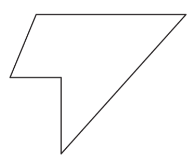How many acute angles are there inside the figure?

1. 5
2. 6
3. 3
4. 4

Solution:(3) 3

Question 4:

Look at the figure below: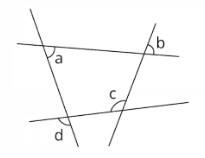__________ and __________ are obtuse angles.

Solution:Since $$\angle c$$ and $$\angle d$$ does not lie within the ruler, it is more than $$90^\circ$$

Hence, they are obtuse angles.

$$\angle c$$ and $$\angle d$$ are obtuse angles.

$$\angle c$$ and $$\angle d$$

Question 5:

Look at the figure below.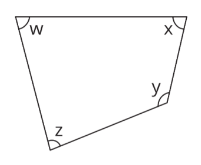Which angle is an obtuse angle?

1. $$\angle w$$
2. $$\angle x$$
3. $$\angle y$$
4. $$\angle z$$

Solution:

After placing the ruler on one line of $$\angle w$$, the second line of $$\angle w$$ lies within the ruler. Hence, $$\angle w$$ is an acute angle. It is the same for $$\angle x$$ and $$\angle z$$After placing the ruler on one line of $$\angle y$$, the second line does not lie within the ruler. Hence, $$\angle y$$ is an obtuse angle.(3) $$\angle y$$

Question 6:

Look at the figure below.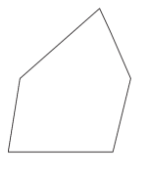How many obtuse angles are there inside the figure?

1. 1
2. 6
3. 3
4. 4

Solution:(3) 3

Question 7:

Look at the figure below.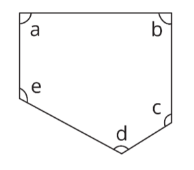__________ and __________ are right angles.

Solution:

After placing the ruler on one line of $$\angle a$$, both the lines forming $$\angle a$$ fit perfectly along the edges of the ruler, making it a right angle. It is the same for $$\angle b$$.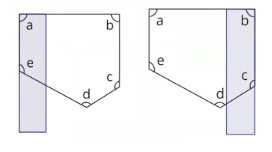$$\angle a$$ and $$\angle b$$ are right angles.

$$\angle c$$, $$\angle d$$ and $$\angle e$$ are obtuse angles.

$$\angle a$$ and $$\angle b$$

Question 8:

Look at the figure below.Which angle is the right angle?

1. $$\angle a$$
2. $$\angle b$$
3. $$\angle c$$
4. $$\angle d$$

Solution:$$\angle a$$ and $$\angle c$$ are obtuse angles.

$$\angle d$$ is an acute angle.

(2) $$\angle b$$

Question 9:

Look at the figure below: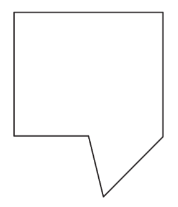How many right angles are there in the figure?

1. 6
2. 2
3. 3
4. 4

Solution:(3) 3

Question 10:

Which of the following figures consists of only 3 right angles inside the figure?

 1)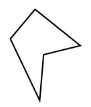2)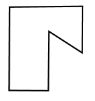3)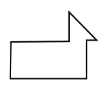4)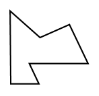Solution:

 1)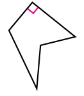2)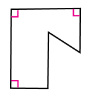3)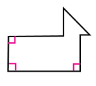4)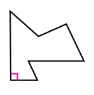(3)

Question 11:

Which of the following figures does not have a right angle inside the figure?

 1)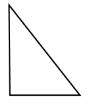2)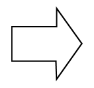3)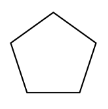4)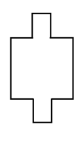Solution:

 1)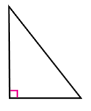2)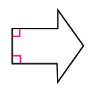3)4)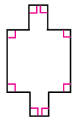(3)

Continue Learning
Volume Money
Mass Fractions
Addition And Subtraction Whole Numbers 1
Whole Numbers 3 Whole Numbers 6
Bar Graphs Angles
Multiplication and Division Length, Mass And Volume
Length Area and Perimeter
Parallel And Perpendicular LinesPrimaryPrimary 1Primary 2Primary 3EnglishMaths
Volume
Money
Mass
Fractions
Whole Numbers 1
Whole Numbers 3
Whole Numbers 6
Bar Graphs
Angles
Multiplication and Division
Length, Mass And Volume
Length
Area and Perimeter
Parallel And Perpendicular LinesSciencePrimary 4Primary 5Primary 6SecondarySecondary 1Secondary 2Secondary 3Secondary 4